# Heat and Mass Transfer of Hydrodynamic Boundary Layer Flow along a Flat Plate with the Influence of Variable Temperature and Viscous Dissipation

Heat and Mass Transfer of Hydrodynamic Boundary Layer Flow along a Flat Plate with the Influence of Variable Temperature and Viscous Dissipation
Department of Mathematical and Physical Sciences, Afe Babalola University, Ado Ekiti 360001, Nigeria

Department of Mathematics, University of Ilorin, Ilorin 240101, Nigeria

Corresponding Author Email:
Page:
441-450
|
DOI:
https://doi.org/10.18280/ijht.390213
5 March 2020
|
Accepted:
14 December 2020
|
Published:
30 April 2021
| Citation

OPEN ACCESS

Abstract:

This paper elucidates heat together with mass transfer through a flat plate and variable temperature as well as dissipative effects. The flow assumptions resulted to steady flow equations which were simplified with appropriate similarity variables. The simplified equations were numerically solved and results are presented both in graphs and tabular form. Effects of physical quantities of interest were presented graphically. The local skin friction is observed to increase because of increase in Schmidt number. Also, increase in Prandtl number is found to boast the local Nusselt number. The behaviour of increase in Prandtl number is found to be unstable within the boundary layer regime while increase in Eckert number produces heat energy within the fluid layers. Finally, the validation of the present problem is done by comparing with previous works and was in perfect agreement.

Keywords:

Eckert number, viscous dissipation, boundary layer, heat and mass transfer

1. Introduction

In recent years, heat together with mass transfer of boundary layer flow gained attention because of its numerous applications in nuclear engineering as well as industries. The results from these studies are of great importance in modeling and simulation. Both thermal and concentration boundary layers develops the moment fluid at specified temperature as well as concentration flows through a surface that is at a different temperature as well as concentration. These are also flow regions over the surface where temperature and concentration variations in the direction normal to the surface are significant. Considering boundary layer flow and effects of heat and mass transfer, velocities as well as velocity gradients are usually assumed to be sufficiently small due to the neglect of effects of the energy on motion as well as and viscous energy dissipation. Hence, this study considers along a flat plate, the effects of viscous dissipation together with convection of heat and mass transfer in boundary layer flow. Gebhart  explained that the heat as a result of viscous dissipation is very crucial when natural convection flow is having a good size, low temperature as well as high gravity. Gebhart and Mollendof  considered viscous dissipative heat transfer on a natural convection flow over a semi-infinite plate

Oosthuizen and David  examined along flat plate effects of viscous dissipation as well as convection of heat transfer, the equation of energy is written by adding the viscous dissipation term. Analysis of mixed convection flow of a viscous dissipating fluid along a vertical flat plate was studied by Aydin et al. . Several researches work on the flow of fluid as well as transfer of heat has been carried out to study effect of viscous thermal dissipation. These studies revealed that fluid flows involving greater Eckert number generates heat because of viscous thermal dissipation and controls the temperature of as well as Eckert number which cannot be zero while investigating convection heat transfer. Brinkman  investigated viscous dissipation on heat transfer in capillary flow. Desale and Pradhan  explains solved the boundary layer equations by considering of viscous dissipation influence through a flat plate in the presence of variable temperature numerically. Oyelami and Dada  studied viscous dissipation influence on heat transfer by natural convection in a porous channel. Tyagi  studied the dissipative fluid in a channel with laminar force convection. Basu et al.  considered the laminar heat transfer by forgone the axial conduction effect in a tube with viscous dissipation. Oyelami and Dada  examined heat as well as mass transfer having effects of viscous dissipation and chemical reactions using Eyring-Powell fluid model.

Alao et al.  analyzed heat together with mass transfer of chemically reacting fluid using spectral relaxation approach. Increase in the parameter of chemical reaction is noticed to decrease the velocity as well as concentration of the fluid. Hayat et al.  elucidate double stratified Powel-Eying liquid chemically reactive flow. Their study concluded that flux as a result of Fourier’s expression is due to the absence of thermal relaxation factor. Mondal et al.  explored MHD mixed convection mass transfer with chemically reacting fluid. Their flow equations were solved numerically and they concluded that increasing the chemical reaction retards the fluid concentration. Falodun et al.  considered chemical reaction effects on non-Newtonian Casson fluid in their study using spectral relaxation approach.

The study of Idowu and Falodun  explored steady free convection flow with chemical reaction using spectral homotopy analysis approach. In the study the chemical reaction parameter is seen to decrease the concentration distribution. Falodun and Omowaye  studied double-diffusive MHD convective flow with chemical reaction using spectral homotopy analysis approach. Their study concluded that increase in chemical reaction parameter increases the local heat transfer rate. Idowu and Falodun  considered the rate of chemical reaction in their study. It was found out in the study that increases in the rate of reaction retards the fluid concentration. The recent work of Waqas  was on analysis of heat transfer of ferromagnetic non-Newtonian liquid. Both homogeneous and heterogeneous chemical reactions are considered in their study. Their study concluded that rate of transportation of heat is improved due to an increased Prandtl number. Mondal et al.  elucidate entropy generation of varied viscosity and thermal radiation with chemical reaction. Their flow equations were solved with spectral quasi-linearization method.

Heat together with mass transfer has become an interesting area of study due to its industrial applications in science as well as engineering such as the exchanger of heat, polymer production, processing of food, petroleum reservoirs etc. Heat is spread when a hot object is in contact with any object. It occurs when an object with $0^{\circ} \mathrm{C}$ falls on object with high temperature, the temperature will be spread on the object with $0^{\circ} \mathrm{C}$ and reduces the temperature of the object at high temperature. Hence, heat has been transferred. Hayat et al.  examined heat generation together with absorption simultaneously with MHD flow of Maxwell nanofluid. Homotopy technique was used to solve their flow equations and it was discovered that thermal radiation enhances both temperature field as well as rate of heat transfer. Kalpana et al.  elucidated impact of temperature dependent viscosity together with thermal conductivity on MHD boundary layer flow of two-phase dusty fluid. Their result shows that there is an enhancement in heat transfer rate as Schmidt, Prandtl and magnetic parameter increases. Raju et al.  elucidates heat together with mass transfer with MHD Casson fluid flow numerically and concluded that increase in heat source parameter enhances rate of heat transfer. Reddy  explored the problem of heat together with mass transfer by considering MHD, thermal radiation as well as chemical reaction. Their flow equations were solved numerically and result revealed that increase in thermal radiation elevates the temperature. Khan et al.  considered heat together with mass transfer of Williamson nanofluid flow. It was concluded in the study that, large values of Prandtl number reduces the heat transport rate. Quwasmeh et al.  examined forced convection heat transfer of Casson fluid using Runge-Kutta-Fehlberg scheme. Their findings revealed that increasing Prandtl number enhances rate of heat transfer.

All the published works discussed above does not consider heat together with mass transfer of hydrodynamic boundary layer flow along a flat plate. Motivated by this assertion and to extend the problem of Desale and Pradhan  to heat together with mass transfer. Hence, this study is necessary because of its various applications in engineering such as in higher-polymer additives in enhancing petroleum pipelines. To the best of our knowledge, no study in literature has considered a problem of this type. The major aim of this paper is to investigate the effects of all flow parameters on local skin friction coefficient, local Nusselt as well as local Sherwood number. We considered the velocity to be high and its effect on the heat transfer has several specialized applications like warmth exchange at high speeds around gas turbine edges or a rocket motors.

2. Governing Equations

Consider a steady flow of a fluid velocity U along a flat plate with the whole surface being held at a uniform temperature $T_{w}$ and concentration Cw. The temperature and concentration of the fluid ahead of the body are $T_{\infty}$ and $C_{\infty}$. For the applications of boundary layer assumptions, Reynold number was assumed to be small enough that the induced magnetic field is neglected. Also, there is no opposition of the magnetic field to the transverse flow. The chemical reaction is of first order as well as the species concentration is assumed to be small so that the effects of Soret-Dufour could be neglected. The viscous dissipation effect is considered to be significant. The physical situation that is modelled in this paper is the flow of Newtonian fluid with constant viscosity together with thermal conductivity over a flat plate (see Figure 1). The temperature situated at the surface of the flat plate is noted to vary. All fluid properties are constant. Boundary layer approximation is valid and under the Bousenesq approximation, the governing equations are as follows:

$\frac{\partial u}{\partial x}+\frac{\partial v}{\partial y}=0$    (1)

$u \frac{\partial u}{\partial x}+v \frac{\partial u}{\partial y}=v \frac{\partial^{2} u}{\partial y^{2}}$      (2)

$u \frac{\partial T}{\partial x}+v \frac{\partial T}{\partial y}=\alpha \frac{\partial^{2} T}{\partial y^{2}}+\frac{\mu}{\rho C_{p}}\left(\frac{\partial u}{\partial y}\right)^{2}$      (3)

$u \frac{\partial C}{\partial x}+v \frac{\partial C}{\partial y}=D \frac{\partial^{2} C}{\partial y^{2}}-k_{c}\left(C-C_{\infty}\right)$     (4)

The boundary conditions are given as

\begin{aligned} u=0, \quad T=T_{w}(x), & C=C_{w}(x) \text { at } y=0 \\ u=U, T=T_{\infty}, C=C_{\infty} \text { as } y \rightarrow \infty \end{aligned}    (5)

where, $u$ and $v$ are velocity components in $x$ and $y$ direction respectively, $v$ is the fluid viscosity, $\alpha$ is the fluid thermal conductivity, $\mathrm{T}$ is the fluid temperature, $\mathrm{C}$ is the fluid concentration, $\mu$ is the coefficient of viscosity, $C_{p}$ is the specific heat at constant pressure, $\rho$ is the fluid density, $D$ is the mass diffusivity, $k_{c}$ is the coefficient of chemical reaction, $T_{\infty}$ is the free stream temperature, $C_{\infty}$ is the free stream concentration, $T_{w}$ is the wall temperature, $C_{w}$ is the wall concentration.

$T_{w}(x)-T_{\infty}=A x^{n}$      (6)

The following similarity variable $\eta$, dimensionless stream function $\psi$, temperature $\theta(\eta)$ as well as concentration $\phi(\eta)$ as

$\eta=y \sqrt{\frac{U}{v x}}, \psi=\sqrt{U v x} f(\eta), \theta(\eta)=\frac{T-T_{\infty}}{T_{w}(x)-T_{\infty}}, \phi(\eta)$

$=\frac{C-C_{\infty}}{C_{w}(x)-C_{\infty}}$     (7)

$u=\frac{\partial \psi}{\partial y}=U f^{\prime}$ and $v=-\frac{\partial \psi}{\partial x}=\frac{1}{2} \sqrt{\frac{U v}{x}}\left(\eta f^{\prime}-f\right)$     (8)

where, $\eta$ is the dimensionless distance, $\psi$ is the stream function, $\theta$ is the dimensionless temperature, and $\phi$ is the dimensionless concentration respectively.

## 1.jpg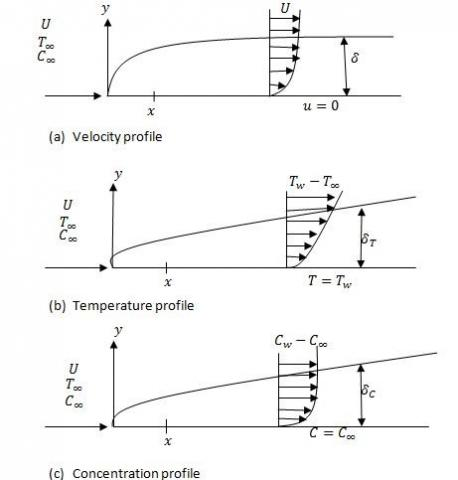Figure 1. Boundary layer of heat together with mass transfer

Note that in this context, prime signifies differentiation with respect to $\eta$.

Eqns. (1) to (5) reduces to the following after applying (6)-(8)

$f^{\prime \prime \prime}+\frac{1}{2} f f^{\prime \prime}=0$      (9)

$\theta^{\prime \prime}+\frac{1}{2} f \theta^{\prime}+E c \operatorname{Pr}\left(f^{\prime \prime}\right)^{2}-n \operatorname{Pr} f^{\prime} \theta=0$      (10)

$\phi^{\prime \prime}+\frac{1}{2} f \phi^{\prime}+S c \lambda f^{\prime} \phi-S c \lambda \phi=0$     (11)

Subject to:

$f^{\prime}(0)=0, f(0)=0, \theta(0)=1, \phi(0)=1$

$f^{\prime}(\infty)=1, \theta^{\prime}(\infty)=0, \phi^{\prime}(\infty)=0 \mid$      (12)

where,

$P r=\frac{v}{\alpha}$  is the dimensionless Prandtl number, $E c=\frac{U^{2}}{C_{p}\left(T_{w}(x)-T_{\infty}\right)}$ is the dimensionless Eckert number, $S c=\frac{v}{D}$ is the is the dimensionless Schmidt number, n is the surface temperature parameter and $\lambda=\frac{l^{2} \gamma}{v}$ is the dimensionless chemical reaction parameter.

The quantities of physical interest representing the rate of heat as well as mass transfer at the surface are $-\theta^{\prime}(0)$ and $-\phi^{\prime}(0)$.

The aim of this work is to investigate how the governing parameters, that is, $\mathrm{Pr}, \mathrm{E} \mathrm{c}, \mathrm{Sc}, \lambda$ and $\mathrm{n}$ influence and $-\theta^{\prime}(0)$ and $-\phi^{\prime}(0)$.

3. Numerical Solution

This section explains the method of approach called spectral homotopy analysis method (SHAM) used in this paper. SHAM is a method of approach that combines the procedure of Chebyshev spedospectral method with the homotopy analysis method (HAM). SHAM helps in decomposing the system of nonlinear Eqns. (9)-(11) into linear equations and Chebyshev spectral collocation method is hereby used to solve the resulting equations. The physical region is first transformed at $[0, \infty)$ to $[-1,1]$using domain truncation technique . Hence, the problem solution is obtained in the interval $\left[0, \eta_{\infty}\right]$ and not $[0, \infty)$ after the following algebraic mapping is applied

$\zeta=\frac{2 \eta}{L}-1, \xi \in[-1,1]$    (13)

To write the boundary conditions in homogeneous form, the following transformation is applied on the transformed equations

$f(\eta )=f(\xi )+{{f}_{0}}(\eta ),\ \theta (\eta )=\theta (\xi )+{{\theta }_{0}}(\eta ),\ \varphi (\eta )=\varphi (\xi )+{{\varphi }_{0}}(\eta )$      (14)

Substituting the above Eq. (14) into (9)-(11) to obtain

$\frac{{{d}^{3}}f}{d{{\xi }^{3}}}+\frac{1}{2}f\frac{{{d}^{2}}f}{d{{\xi }^{2}}}+{{a}_{1}}f+{{a}_{2}}\frac{{{d}^{2}}f}{d{{\xi }^{2}}}={{H}_{1}}(\eta )$     (15)

\begin{align} & \frac{{{d}^{2}}\theta }{d{{\xi }^{2}}}+\frac{1}{2}f\frac{d\theta }{d\xi }+{{b}_{1}}f+{{b}_{2}}\frac{d\theta }{d\xi }+EcPr\frac{{{d}^{2}}f}{d{{\xi }^{2}}}\frac{{{d}^{2}}f}{d{{\xi }^{2}}} \\ & +{{b}_{3}}\frac{{{d}^{2}}f}{d{{\xi }^{2}}}+{{b}_{4}}\frac{df}{d\xi }+{{b}_{5}}\theta ={{H}_{2}}(\eta ) \\\end{align}      (16)

\begin{align} & \frac{{{d}^{2}}\varphi }{d{{\xi }^{2}}}+\frac{1}{2}f\frac{d\varphi }{d\xi }+{{c}_{1}}f+{{c}_{2}}\frac{d\varphi }{d\xi }+Sc\lambda \varphi \frac{df}{d\xi } \\ & +{{c}_{3}}\frac{df}{d\xi }+{{c}_{4}}\varphi -Sc\lambda \varphi ={{H}_{3}}(\eta ) \\\end{align}     (17)

where,

${{a}_{1}}=\frac{1}{2}\frac{{{d}^{2}}{{f}_{0}}}{d{{\eta }^{2}}},\ {{a}_{2}}=\frac{1}{2}{{f}_{0}}\ ,{{H}_{1}}(\eta )=-\frac{{{d}^{3}}{{f}_{0}}}{d{{\eta }^{3}}}-\frac{1}{2}{{f}_{0}}\frac{{{d}^{2}}{{f}_{0}}}{d{{\eta }^{2}}}$

${{b}_{1}}=\frac{d{{\theta }_{0}}}{d\eta },\ {{b}_{2}}=\frac{1}{2}{{f}_{0}},\ {{b}_{3}}=2EcPr\frac{{{d}^{2}}{{f}_{0}}}{d{{\eta }^{2}}},\ {{b}_{4}}=-nPr{{\theta }_{0}},\ {{b}_{5}}=-nPr\frac{d{{f}_{0}}}{d\eta }$

${{H}_{2}}=-\frac{{{d}^{2}}{{\theta }_{0}}}{d{{\eta }^{2}}}-\frac{1}{2}{{f}_{0}}\frac{d{{\theta }_{0}}}{d\eta }-EcPr\frac{{{d}^{2}}{{f}_{0}}}{d{{\eta }^{2}}}\frac{{{d}^{2}}{{f}_{0}}}{d{{\eta }^{2}}}+nPr{{\theta }_{0}}\frac{d{{f}_{0}}}{d\eta }$

${{c}_{1}}=\frac{1}{2}\frac{d\varphi }{d\eta },\ {{c}_{2}}=\frac{1}{2}{{f}_{0}},\ {{c}_{3}}=Sc\lambda {{\varphi }_{0}},\ {{c}_{4}}=Sc\lambda \frac{d{{f}_{0}}}{d\eta }$

${{H}_{3}}=-\frac{{{d}^{2}}{{\varphi }_{0}}}{d{{\eta }^{2}}}-\frac{1}{2}{{f}_{0}}{{\varphi }_{0}}-Sc\lambda \varphi \frac{d{{f}_{0}}}{d\eta }+Sc\lambda {{\varphi }_{0}}$

Eqns. (15)-(17) are nonlinear equations. In the application of SHAM, the nonlinear equations are decomposed into linear and nonlinear parts. It should be noted that the derivatives of $f, \theta$ and $\phi$ are with respect to $\xi$ defined by:

$\frac{d}{d\xi }=\frac{2}{L}\frac{d}{d\eta }$      (18)

An initial guess is taken with reference to the conditions in (12) as the following functions

${{f}_{0}}(\eta )={{e}^{-\eta }}-1,\ {{\theta }_{0}}(\eta )={{\varphi }_{(}}\eta )={{e}^{-\eta }}$      (19)

The non-homogeneous linear part of Eqns. (15)-(17) are decomposed from the nonlinear part as the following equations

$\frac{{{d}^{3}}f}{d{{\xi }^{3}}}+{{a}_{1}}f+{{a}_{2}}\frac{{{d}^{2}}f}{d{{\xi }^{2}}}={{H}_{1}}(\eta )$     (20)

\begin{align} & \frac{{{d}^{2}}\theta }{d{{\xi }^{2}}}+{{b}_{1}}f+{{b}_{2}}\frac{d\theta }{d\xi }+{{b}_{3}}\frac{{{d}^{2}}f}{d{{\xi }^{2}}} \\ & +{{b}_{4}}\frac{df}{d\xi }+{{b}_{5}}\theta ={{H}_{2}}(\eta ) \\\end{align}      （21）

\begin{align} & \frac{{{d}^{2}}\varphi }{d{{\xi }^{2}}}+{{c}_{1}}f+{{c}_{2}}\frac{d\varphi }{d\xi }+{{c}_{3}}\frac{df}{d\xi } \\ & +{{c}_{4}}\varphi -Sc\lambda \varphi ={{H}_{3}}(\eta ) \\\end{align}      (22)

Subject to:

${{f}_{l}}(-1)=f{{'}_{l}}(1)=f{{'}_{l}}(1)=0,{{\theta }_{l}}(-1)={{\theta }_{l}}(1)=0,{{\varphi }_{l}}(-1)={{\varphi }_{l}}(1)=0$      (23)

The boundary conditions (23) are chosen to be all equals to zero with reference to the transformed domain [-1, 1], to be able to implement the linear part SHAM solution. The Chebyshev pseudospectral method is applied on Eqns. (20)-(22). The Chebyshev nodes in the transformed domain [-1, 1] are defined by the Gauss-Lobatto collocation points  given by

${{\xi }_{J}}=cos\left( \frac{\pi j}{N} \right)$     (24)

provided $j=0,1,...N$ and $N+1$ is the number of collocation points. The unknown functions ${{f}_{l}}(\xi ),{{\theta }_{l}}(\xi )$ and ${{\varphi }_{l}}(\xi )$ are approximated by the use of Lagrange form of interpolating polynomial which interpolates the unknown functions ${{f}_{l}}(\xi ),{{\theta }_{l}}(\xi )$ and ${{\varphi }_{l}}(\xi )$ at the Gauss-Lobatto collocation points as defined in Eq. (24).

4. Discussion of Results

This study elucidates solution to the flow of hydrodynamic boundary layer of heat together with mass transfer and variable temperature using SHAM. The idea of SHAM is to decompose nonlinear differential equations by combining the concept of Chebyshev pseudospectral and homotopy analysis method together. The default values of parameters used in this paper are: $E c=0.01, P r=0.71, n=1.0, S c=0.61, \lambda=2.0$. Hence, all graphs and tables correspond to these values unless or otherwise stated. This paper describes the law of change in temperature which can be explained using the Newton’s law of cooling. This law states that the rate of heat exchange between an object and its surroundings is proportional to the difference in temperature between the object and the surroundings. Hence, there is a temperature difference of fluid particles within the flat plate surface which is considered subject to the viscous dissipation in this paper.

Figures 2-9 depict the effect of flow parameters on velocity, temperature as well as concentration distributions together with the effect of pertinent flow parameters on the three engineering quantities of interest, that is, local skin friction coefficient, local Nusselt and Sherwood number.

Table 1. Variations of Pr and Ec on the local skin friction, local Nusselt as well as Sherwood number

 Pr Ec=0.1 Ec=0.3 Ec=0.5 -Cf Nux Shx 0.71 0.4623 1.4411 0.7176 1.00 0.3774 1.9901 0.7189 7.00 0.3484 2.1117 0.7909

Table 1 shows the variation of Ec and Pr on the local skin friction coefficient, local Nusselt and Sherwood number. A reduction on the skin friction coefficient is noticed as Pr increases. Also, an increase in Nusselt and Sherwood number is noticed as the values Pr and Ec varies. The outcomes in Table 1 are valid because a higher value of Ec produces heat energy which speed up the rate of heat transport by enhancing the local Nusselt number.

Table 2. Computational values of Sherwood number for different values of Sc

 Sc Shx 0.22 0.6794 0.61 0.7730 1.00 0.8809

Table 2 presents the computational values of Sherwood number as Schmidt number increases. Sc denotes the rate of mass transfer parameter. It is found out that increase in Sc leads to increase in the Sherwood number. This is true because the significant of Sc is to aid the rate of mass transport within the concentration boundary layer. The absence of Sc, that is Sc=0 means rate of mass transfer is negligible.

Table 3. Computational values of local Nusselt number for different values of Pr

 Pr Nux 0.71 0.8252 1.00 0.9608 7.00 2.5119

Table 3 elucidates the computational values of Nusselt number as Pr increases. A large number of Pr is seen to increase the rate of heat transfer. Large Pr indicates greater viscosities which add more temperature to the fluid motion by increasing the rate of heat transfer. It is because fluids with a large value of Pr acquires much viscosities which serve to degenerate the velocity and temperature profile. This in turn lead to decrease in the momentum and thermal boundary layer thickness. Small values of Pr, $($ i. $e P r<1)$, the fluid is highly conducive.

## 21.jpg## 22.jpg## 23.jpg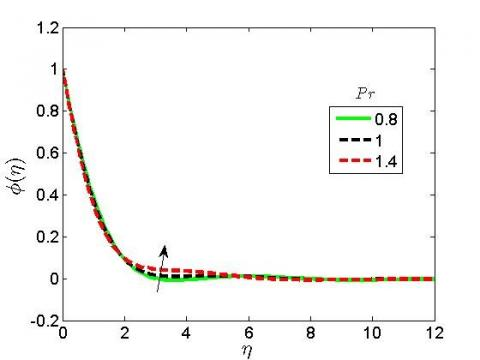Figure 2. Pr effect on velocity, temperature as well as concentration distributions

Figure 2 shows that Prandtl number (Pr) boast the velocity, temperature as well as concentration distributions. Pr is division of the fluid viscosity to thermal conductivity. Hence, if the viscosity is more than the thermal conductivity, there is going to be a higher value of Pr but if the thermal conductivity is more than the viscosity, there is going to be a low value of Pr. Physically, small values of Pr, that is, Pr<1 means that the fluid is highly conducive.

Table 4 explains the comparison of the present result from SHAM with that of Desale and Pradhan  results from Keller box method in the absence of the species equation. The two results were in close agreement. This implies that the present result is valid for the problem of heat together with mass transfer along a flat plate.

Table 4. Comparison of the present study with Desale and Pradhan  when Pr=0.7 for variable temperature as well as the absence of species equation

 Desale and Pradhan (2015) Keller Box Method n Ec=0.1 Ec=0.3 Ec=0.5 Ec=0.7 1 0.471081 $0.45243$ $0.433778$ $0.415127$ 2 $0.576896$ $0.56069$ $0.544484$ $0.528278$ 3 $0.654579$ $0.639866$ $0.625153$ $0.61044$ 4 $0.717506$ $0.703844$ $0.690181$ $0.676519$ Present study, Spectral homotopy analysis approach n $E_{C}=0.1$ $E_{C}=0.1$ $E_{C}=0.1$ $E_{C}=0.1$ 1 $0.471079$ $0.471079$ $0.471079$ $0.471079$ 2 $0.576889$ $0.576889$ $0.576889$ $0.576889$ 3 $0.654577$ $0.654577$ $0.654577$ $0.654577$ 4 $0.717502$ $0.717502$ $0.717502$ $0.717502$

## 31.jpg## 32.jpg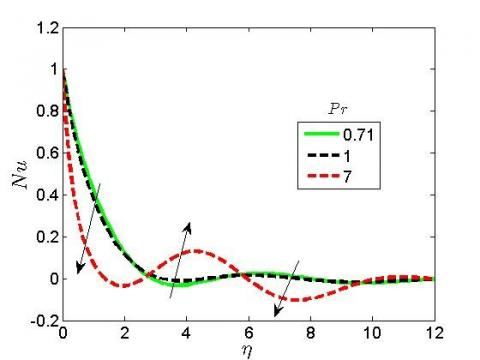## 33.jpg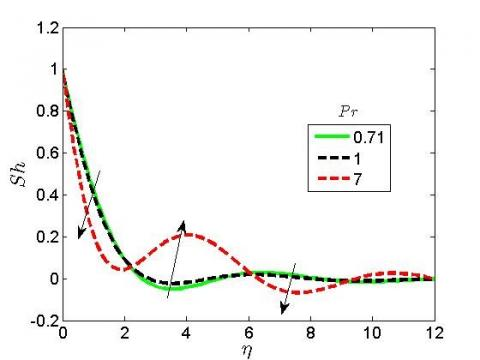Figure 3. Pr effect on local skin friction coefficient, local Nusselt as well as Sherwood numbers

As shown in Figure 3, increase in the values of Pr lower the skin friction coefficient but has an unstable behaviour on the local Nusselt as well as Sherwood number. This shows that the behaviour of increase in Pr on the rate of heat together with mass transfer is not stable. Hence, it results to a zig-zag manner of the thermal boundary layer thickness.

## 41.jpg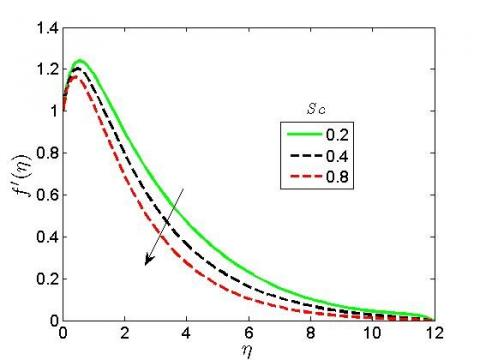## 42.jpg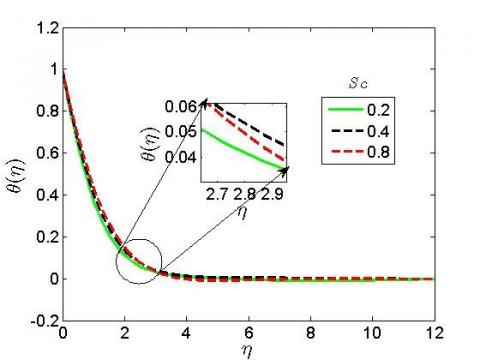## 43.jpg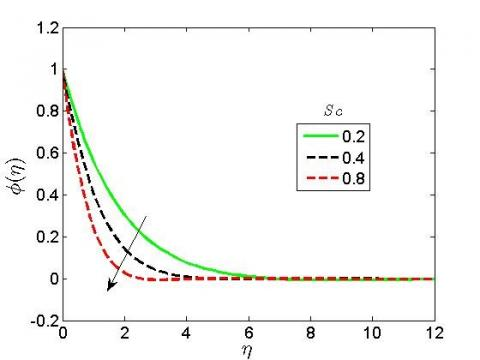Figure 4. Sc effect on velocity, temperature and concentration distributions

Figure 4 explains the behaviour of Schmidt number (Sc) on the boundary layer flow regime. Increase in the values of Sc is seen to reduce the velocity as well as concentration distribution. Sc is responsible for the control of rate of mass transfer effect. When it is zero, that is Sc=0, it means that there is no specie equation and transfer of mass does not take place in the problem because Sc is the division of momentum diffusivity to mass diffusivity. In Figure 4, increases in Sc decreases the velocity and concentration distributions because of buoyancy effects as a result of concentration which often decelerate velocity of the fluid. Increase in Sc is seen to increase the rate of heat transfer as the fluid temperature increases. Physically, Sc  explains the relationship between the thickness of hydrodynamic layer as well as mass transfer layer. Sc is equivalent to Pr in the process of heat transfer. When Sc=1, this means that both momentum and mass transfer are similar. In fact, the two process may likely coincide. Liquids mass diffusion increases with temperature. For example, diffusion coefficient for ethanol in water Dethanol, water $=1.6 \times 10^{-9}$ whose $S c=540$.

## 51.jpgFigure 5. Sc effect on local skin friction coefficient, local Nusselt as well as Sherwood number

Figure 5 shows an irregular behaviour of Sc on the local skin friction, local Nusselt as well as Sherwood number. Increase in Sc is seen to have great effect on skin friction coefficient, local Nusselt as well as Sherwood number. This shows that rate of momentum, heat together with mass transfer is irregular.

In Figure 6, increase in the viscous dissipation term (Eckert number, (Ec)) is seen to decrease the velocity, temperature as well concentration distributions. Physically, increase in Eckert number lead to increase in heat energy within the fluid layer. Due to decrease in the flow regimes, the heat energy produced by Eckert number is not stored and there is no existence of frictional heating.

## 61.jpg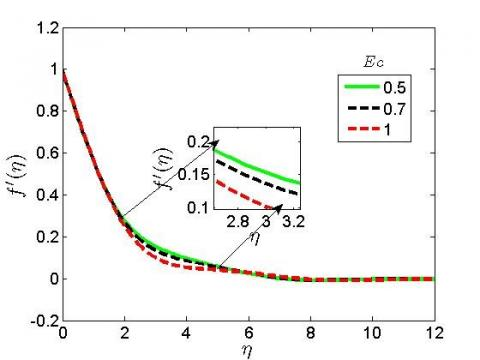## 62.jpg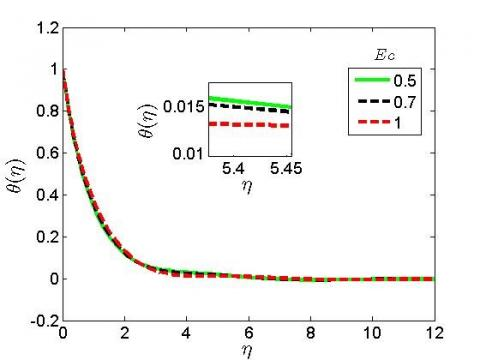## 63.jpg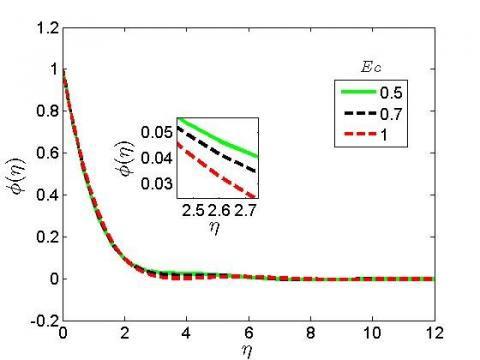Figure 6. Ec effect on velocity, temperature as well as concentration distributions

Figure 7 elucidates influence of Ec on the physical quantity of engineering interest. An irregular behaviour of local skin friction coefficient, local Nusselt as well as Sherwood number is seen in Figure 7 because of in Ec. Obviously in Figure 7, there is a spontaneous behaviour in the momentum, heat together with mass transfer rate. This could be as a result of the presence of surface temperature. It is noticed that when the surface temperature n=0, increase in Ec causes a rise in velocity as well as the temperature.

Figure 8 shows that increase in the chemical reaction parameter (\lambda) results to enhancement in the momentum and mass boundary layer by increasing the fluid velocity and concentration. Reaction rate is subject to the concentration of species itself. Chemical reaction takes place when there is a mixture of fluid particles with the atmosphere. A new compound will be produced due to heterogeneous reactions which takes place between two different states of matters. The graphical results as shown in Figure 8 means that the chemical reaction is not destructive $\lambda<0$ which strengthens the buoyancy effect as a result of concentration gradients.

## 71.jpg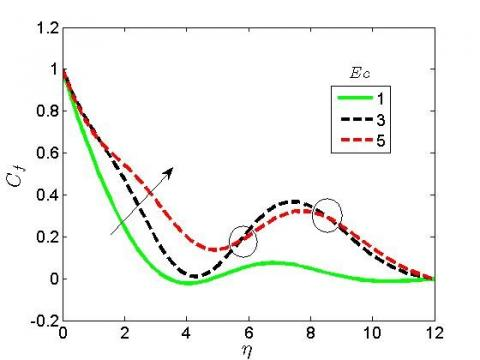## 72.jpg## 73.jpg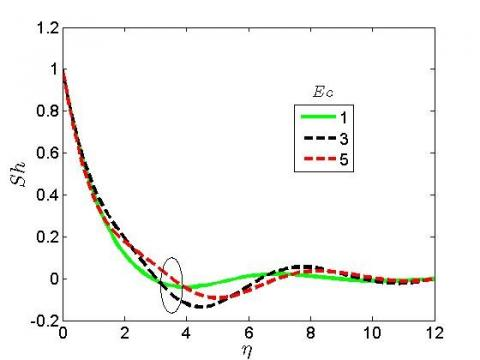Figure 7. Ec effect on local skin friction coefficient, local Nusselt as well as Sherwood number

## 81.jpg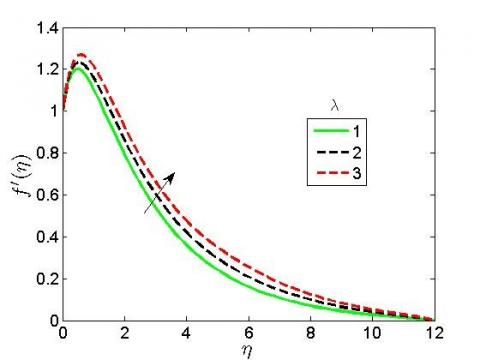## 82.jpg## 83.jpgFigure 8. $\lambda$ effect on velocity, temperature as well as concentration distributions

Figure 9 presents increase in $\lambda$ reduces the local skin friction coefficient, local Nusselt as well as Sherwood number. This implies that momentum, heat together with mass transfer rate decreases within the fluid layers.

## 91.jpg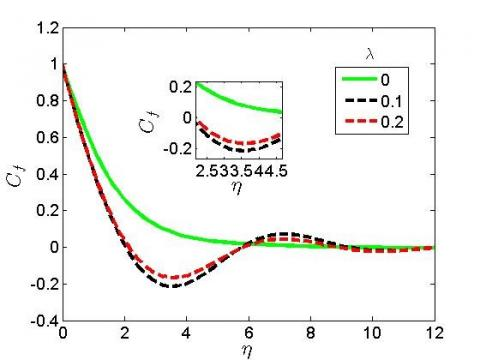## 92.jpg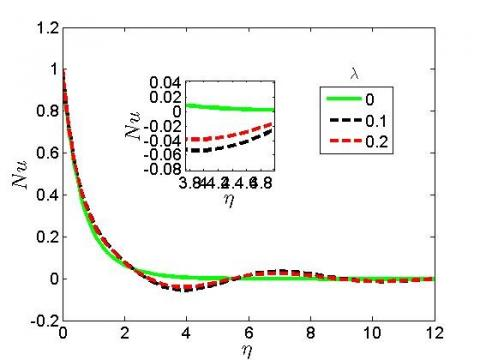## 93.jpg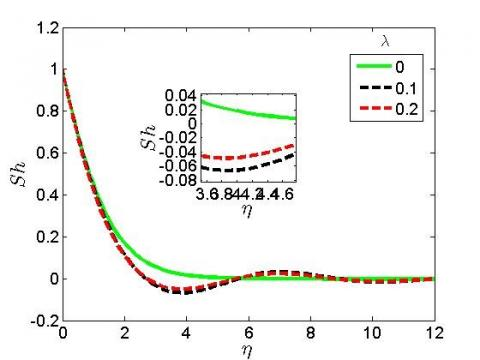Figure 9. $\lambda$ effect on local skin friction coefficient, local Nusselt as well as Sherwood numbers

5. Conclusion

This paper elucidates the problem of heat together with mass transfer flow of boundary layer through a flat plate. The flow of fluid particles within the flat plate is assumed to be laminar. In this case, the fluid flows on its own streamline path with no mass transfer between the adjacent fluid layers. The shear is because of molecular action. The laminar flow conditions are assumed in this study because of low Reynolds number. The flow equations were successfully solved numerically using spectral homotopy analysis method. The results in this study using SHAM is in good agreement with methods in literature. Graphical results for velocity, temperature, concentration, local skin friction coefficient, local Nusselt as well as local Sherwood number by varying pertinent flow parameters like Prandtl number, Schmidt number, Eckert number as well as chemical reaction parameter are presented. The main findings of this paper are:

1. Increase in Pr and Ec brings increase to both local skin friction, local Nusselt as well as Sherwood number;
2. Increase in Pr brings irregular behaviour to the local skin friction coefficient, local Nusselt as well as Sherwood number;
3. The velocity as well as concentration profiles drastically decreases with increase in Sc. A reverse case is observed on the temperature distribution as increase in Sc enhances the thermal boundary layer thickness; and
4. As the chemical reaction parameter increases, an increase in velocity and concentration profiles is observed.

The SHAM is more efficient because of its flexibility in the choice of linear operators in comparison to various methods in literature reviewed in this study. Hence, the choice of SHAM is because of its elegance and accuracy. The problem considered in this paper will be helpful in oil-pipeline friction reduction, surfactant applications to large-scale heating together with cooling systems. It will also be useful in high-polymer additives to enhance flow in petroleum pipe-lines which is very useful for commercial purposes.

Nomenclature
 u,v velocity components in x and y-direction U wall velocity C fluid concentration T fluid temperature Tw wall temperature Cw wall concentration $T_{\infty}$ free stream temperature $C_{\infty}$ free stream concentration D mass diffusivity $C_{p}$ specific heat at constant pressure $\alpha$ thermal conductivity $\mu$ coefficient of viscosity $\rho$ fluid density $\eta$ dimensionless distant $\theta$ dimensionless temperature $\phi$ dimensionless concentration
References

 Gebhart B. (1962). Effects of viscous dissipation in natural convection. Journal of Fluid Mechanics, 14(2): 225-235.

 Gebhart, B., Mollendorf, J. (1969). Viscous dissipation in external natural convection flows. Journal of Fluid Mechanics, 38(1): 97-107. https://doi.org/10.1017/S0022112069000061

 Patrick Oosthuizen, H., David, N. (1999). An Introduction to Convective Heat Transfer Analysis. WCB McGraw-Hill, Singapore.

 Aydin, O., Kaya, A. (2007). Mixed convection of a viscous dissipating fluid about a vertical flat plate. Applied Mathematical Modelling, 31(5): 843-853. https://doi.org/10.1016/j.apm.2005.12.015

 Brinkman, H.C. (1951). Heat transfer in capillary flow I. Applied Science Research, 2: 120-124. http://dx.doi.org/10.1007/BF00411976

 Oyelami, F.H., Dada, M.S. (2018). Unsteady magnetohydrodynamic flow of some non-Newtonian fluids with slip in a porous channel. International Journal of Heat and Technology, 36(2): 709-713. https://doi.org/10.18280/ijht.360237

 Tyagi, V.P. (1996). Laminar forced convection of a dissipative fluid in a channel. Journal of Heat Transfer 88(2): 161-169. https://doi.org/10.1115/1.3691501

 Basu, T., Roy, D.N. (1985). Laminar heat transfer in a tube with viscous dissipation. Int. J. Heat Mass Transfer, 28(3): 699-701. https://doi.org/10.1016/0017-9310(85)90191-7

 Desale, S., Pradhan, V.H. (2015). Numerical solution of boundary layer flow equation with viscous dissipation effect along a flat plate with variable temperature. Procedia Engineering, 127: 846-853. https://doi.org/10.1016/j.proeng.2015.11.421

 Oyelami, F.H., Dada, M.S. (2018). Numerical analysis of non-Newtonian fluid in a non-Darcy porous channel. Modelling, Measurement and Control B, 87(2): 83-91. https://doi.org/10.18280/mmc_b.870204

 Alao, F.I., Fagbade, A.I., Falodun, B.O. (2016). Effects of thermal radiation, Soret and Dufour on an unsteady heat and mass transfer flow of a chemically reacting fluid past a semi-infinite vertical plate with viscous dissipation. Journal of the Nigerian Mathematical Society, 35(1): 142-158. https://doi.org/10.1016/j.jnnms.2016.01.002

 Hayat, T., Zubair M., Waqas, M., Alsaedi, A., Ayub, M. (2017). On doubly stratified chemically reactive flow of Powell–Eyring liquid subject to non-Fourier heat flux theory. Results in Physics, 7: 99-106 https://doi.org/10.1016/j.rinp.2016.12.003

 Mondal, H., Dulal, P., Sewli, C., Precious, S. (2018). Thermophoresis and Soret-Dufour on MHD mixed convection mass transfer over an inclined plate with non-uniform heat source/sink and chemical reaction. Ain Shams Engineering Journal, 9(4): 2111-2121. https://doi.org/10.1016/j.asej.2016.10.015

 Falodun, B.O., Onwubuoya, C., Awoniran, F.H. (2018). Magnetohydrodynamics (MHD) heat and mass transfer of casson fluid flow past a semi-infinite vertical plate with thermophoresis effect: Spectral relaxation analysis. Defect and Diffusion Forum Submitted, 389: 18-35. http://dx.doi.org/10.4028/www.scientific.net/DDF.389.18

 Idowu, A.S., Falodun, B.O. (2018). Influence of magnetic field and thermal radiation on steady free convective flow in a porous medium. Nigerian Journal of Technological Development, 15(3): 84-97. https://doi.org/10.4314/njtd.v15i3.3

 Falodun, B.O., Omowaye, A. J. (2019). Double-diffusive MHD convective flow of heat and mass transfer over a stretching sheet embedded in a thermally-stratified porous medium. World Journal of Engineering, 16(6): 1-13. http://dx.doi.org/10.1108/WJE-09-2018-0306

 Idowu, A.S., Falodun, B.O. (2019). Soret–Dufour effects on MHD heat and mass transfer of Walter’s-B viscoelastic fluid over a semi-infinite vertical plate: Spectral relaxation analysis. Journal of Taibah University for Science, 13(1): 49-62. http://dx.doi.org/10.1080/16583655.2018.1523527

 Waqas, M. (2020). A mathematical and computational framework for heat transfer analysis of ferromagnetic non-Newtonian liquid subjected to heterogeneous and homogeneous reactions. Journal of Magnetism and Magnetic Materials, 493: 165646. http://dx.doi.org/10.1016/j.jmmm.2019.165646

 Hiranmoy, M., Shweta, M., Prabir, K., Precious, S. (2020). Entropy generation of variable viscosity and thermal radiation on magneto nanofluid flow with dusty fluid. Journal of Applied Computational Mechanics, 6(1): 171-182. http://dx.doi.org/10.22055/JACM.2019.28273.1473

 Tasawar, H., Sajid, Q., Sabir, A., Ahmed, A. (2017). Simultaneous effects of heat generation/absorption and thermal radiation in magnetohydrodynamics (MHD) flow of Maxwell nanofluid towards a stretched surface. Results in Physics, 7: 562-573. https://doi.org/10.1016/j.rinp.2016.12.009

 Kalpana, G., Madhura, K.R., Ramesh, B. (2019). Impact of temperature-dependant viscosity and thermal conductivity on MHD boundary layer flow of two-phase dusty fluid through permeable medium. Engineering Science and Technology, an International Journal, 22(2): 416-427. https://doi.org/10.1016/j.jestch.2018.10.009

 Raju, C.S.K., Sandeep, N., Sugunamma, V., Jayachandra, M., Ramana, J.V. (2016). Heat and mass transfer in magnetohydrodynamic Casson fluid over an exponentially permeable stretching surface. Engineering Science and Technology, an International Journal, 19(1): 45-52. https://doi.org/10.1016/j.jestch.2015.05.010

 Reddy, P.B.A. (2016), Magnetohydrodynamic flow of a Casson fluid over an exponentially inclined permeable stretching surface with thermal radiation and chemical reaction. Ain Shams Engineering Journal, 7(2): 593-602. https://doi.org/10.1016/j.asej.2015.12.010

 Khan, M., Malik, M.Y., Salahuddin, T., Hussian, A. (2018). Heat and mass transfer of Williamson nanofluid flow yield by an inclined Lorentz force over a nonlinear stretching sheet. Results in Physics, 8: 862-868. https://doi.org/10.1016/j.rinp.2018.01.005

 Qawasmeh, B.R., Mohammad, A, Sameer, A. (2019). Forced convection heat transfer of Casson fluid in non-Darcy porous Media. Advances in Mechanical Engineering, 11(1): 1-10. https://doi.org/10.1177/1687814018819906

 Sibanda, P, Motsa, S.S., Makukula, Z.G. (2012). A spectral-homotopy analysis method for heat transfer flow of a third grade fluid between parallel plates. Int. J. Numerical Methods Heat Fluid Flow, 22(1): 4-23. https://doi.org/10.1108/09615531211188766

 Trefethen, L.N. (2000). Spectral Methods in MATLAB. SIAM. http://dx.doi.org/10.1137/1.9780898719598